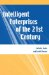# Restrictions and Considerations

When you are considering dynamic SQL over static SQL or vice versa, you should also understand that dynamic SQL uses only statements that can be dynamically prepared. Hence, dynamic SQL must be any of the following statements shown in Table 7.1

##### Table 7.1. Statements That Can Be Dynamically Prepared

Statement

LUW

iSeries

zSeries

ALLOCATE CURSOR

N

NA

Y

ALTER

Y

Y

Y

ASSOCIATE LOCATOR

N

NA

Y

CALL

Y

Y

N

CLOSE

Y

N

N

COMMENT

Y

Y

Y

COMMIT

Y

Y

Y

CONNECT

Y

N

N

CREATE

Y

Y

Y

DECLARE GLOBAL TEMPORARY TABLE

Y

Y

Y

DELETE

Y

Y

Y

DESCRIBE

N

N

N

DISCONNECT

N

N

NA

DROP

Y

Y

Y

EXECUTE

N

N

N

EXECUTE IMMEDIATE

Y

N

N

EXPLAIN

Y

NA

Y

FETCH

N

N

N

FLUSH EVENT MONITOR

Y

NA

NA

FREE LOCATOR

Y

Y

Y

GET DIAGNOSTICS

N

N

N

GRANT

Y

Y

Y

HOLD LOCATOR

N

Y

Y

INSERT

Y

Y

Y

LABEL

N

Y

Y

LOCK TABLE

Y

Y

Y

OPEN

N

N

N

PREPARE

N

N

N

REFRESH TABLE

Y

Y

Y

RELEASE (Connection)

N

N

N

RELEASE SAVEPOINT

Y

Y

Y

RENAME INDEX

Y

Y

NA

RENAME TABLE

Y

Y

Y

RENAME TABLESPACE

Y

Y

Y

ROLLBACK

Y

Y

Y

SAVEPOINT

Y

Y

Y

SELECT (statement)

Y

Y

Y

SELECT INTO

Y

N

N

SET CONNECTION

Y

N

N

SET CURRENT APPLICATION ENCODING SCHEME

N

NA

N

SET CURRENT DEFAULT TRANSFORM GROUP

Y

NA

NA

SET CURRENT DEGREE

Y

NA

Y

SET CURRENT EXPLAIN MODE

Y

NA

NA

SET CURRENT EXPLAIN SNAPSHOT

Y

NA

NA

SET CURRENT LOCALE LC_CTYPE

N

NA

Y

SET CURRENT MAINTAINED TABLE TYPES FOR OPTIMIZATION

Y

NA

Y

SET CURRENT OPTIMIZATION HINT

N

NA

Y

SET CURRENT PACKAGE PATH

N

NA

N

SET CURRENT PACKAGESET

N

NA

N

SET CURRENT PRECISION

N

NA

Y

SET CURRENT QUERY OPTIMIZATION

Y

NA

NA

SET CURRENT REFRESH AGE

Y

NA

Y

SET CURRENT RULES

N

NA

Y

SET CURRENT SQLID

N

NA

Y

N

Y

Y

SET EVENT MONITOR STATE

Y

NA

NA

SET INTEGRITY

Y

NA

NA

SET PASSTHRU

Y

NA

NA

SET PATH

Y

Y

Y

SET RESULT SETS

NA

N

NA

SET SCHEMA

Y

Y

Y

SET SERVER OPTION

Y

NA

NA

SET TRANSACTION

N

Y

NA

SIGNAL SQLSTATE

N

N

N

UPDATE

Y

Y

Y

VALUES INTO

N

Y

N

Different methods are available for you to work with dynamic SQL in SQL procedures, and the following subsections will discuss each in turn.

Note

On zSeries, some statements can be dynamically prepared only if DYNAMICRULES run behavior is implicitly or explicitly specified. Refer to the corresponding SQL Reference manual for details.Intelligent Enterprises of the 21st Century
ISBN: N/A
EAN: 2147483647
Year: 2003
Pages: 205